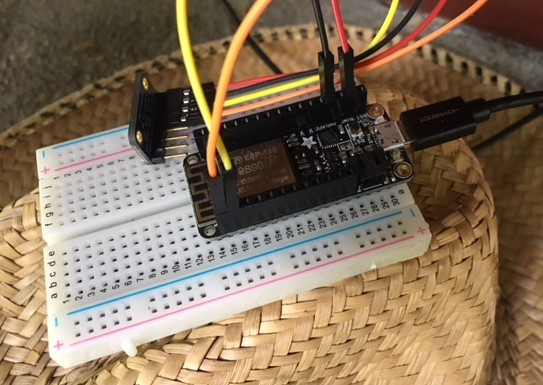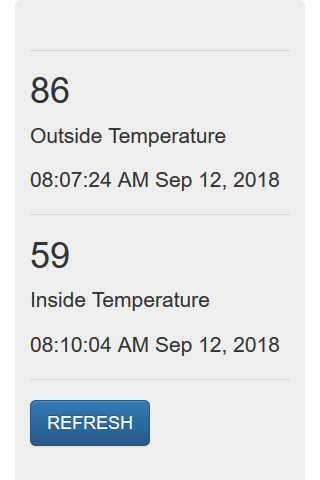## Solving Two Equations for Two Unknowns and a Statics Problem with SymPy and Python

SymPy (http://www.sympy.org) is a Python library for symbolic math.

In symbolic math, symbols represent mathematical expressions. In a numerical calculation, the value of pi is stored as an estimate of pi, a floating point number close to 3.14.... In a symbolic math expression, the value of …

## Solving Equations and Writing Expressions with SymPy and Python

SymPy (http://www.sympy.org) is a Python library for symbolic math.

In symbolic math, symbols are used to represent mathematical expressions. An example of a symbolic math expression is below:

$$x^{2} + y^{2} = z$$

In the expression above, we have the variables $x$, $y$ and $z$.

If we …

## Building an IoT Server with flask and Python - Part 6 - upload code to ESP8266-based WiFi weather stations

Date Series Part 6 of Building an IoT Server with Flask and Python Tags python / flask / IoT / sensorThis is the sixth part of a series of posts about building an Internet of Things (IoT) server with flask, Python and ESP8266 microcontrollers. In this post, we'll add some code to our ESP8266-based weather stations. The code we upload to the ESP8266 microcontrollers programs the WiFi weather stations to …

## Building an IoT Server with flask and Python - Part 5 Adding a DatabaseThis is the fifth part of a series of posts about building an Internet of Things (IoT) server with flask, Python and ESP8266 microcontrollers. In this post, we'll add a sqlite3 database to our flask IoT server to store all the temperature data points that come in from our ESP8266-based …

## Building an IoT Server with flask and Python - Part 4 Validation and Timestamps

This is the fourth part of a series of posts about building an Internet of Things (IoT) server with flask, Python and ESP8266 microcontrollers. In the last post of the series, we reviewed how to build a web API with flask which accepts temperature measurements. In this post, we'll build …